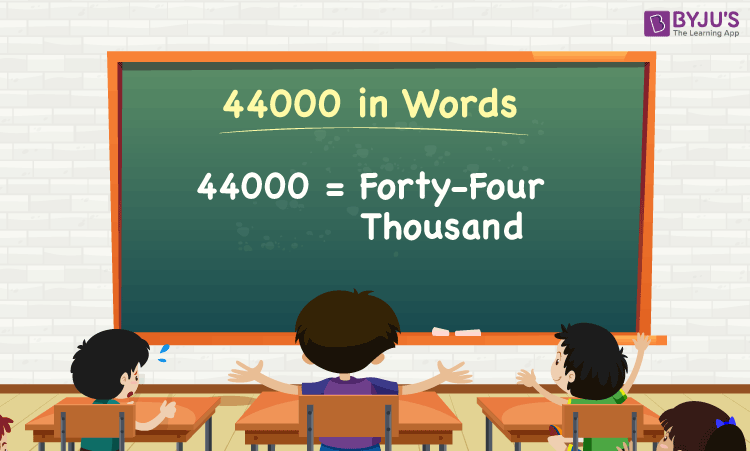# 44000 in Words

44000 in words is Forty-four Thousand. For example, if you earned Rs. 44000 in a month, you can write, “I have earned Rs. Forty-four Thousand in a month”. In general, the number name of any number can be written using the ones, tens, hundreds, and thousands place of a number. Thus, the place value chart is useful for writing the number 44000 in words.

 44000 in Words: Forty-four Thousand

In this article, we will learn how to write 44000 in words, and look at the solved examples in detail.

## How to Write 44000 in Words?To write the number name of 44000, first, we have to determine the place value of each digit of the given number 44000. Hence, for the number 44000,

1’s place of 44000 is 0

10’s place of 44000 is 0.

100’s place of 44000 is 0.

1000’s place of 44000 is 44.

The following chart represents the place value of the number 44000:

 Thousands Hundreds Tens Ones 44 0 0 0

Therefore, the number 44000 in words is forty-four Thousand.

### Examples

Example 1:

Find the value of 24000 + 20000. Describe the value in words.

Solution:

Given expression: 24000 + 20000

⇒ 24000 + 20000 = 44000

So, the value of 24000 + 20000 is 44000.

Hence, 44000 in words is forty-four thousand.

Example 2:

Express the value of forty-four thousand minus four thousand in words.

Solution:

Forty-four Thousand = 44000

Four thousand = 4000

Forty-four Thousand minus four thousand = 44000 – 4000 = 40000

Hence, the value of forty-four thousand minus four thousand is forty thousand in words.

Stay tuned to BYJU’S – The Learning App, and download the app to learn all Maths concepts quickly.

## Frequently Asked Questions on 44000 in Words

Q1

### Write 44000 in words.

44000 in words is forty-four thousand.

Q2

### Express the value 44500 – 500 in words.

Simplifying 44500 – 500, we get 44000. Hence, 44000 in words is forty-four thousand.

Q3

### How to write forty-four thousand in numbers?

Forty-four thousand in numbers is 44000.

Q4

### Is the number 44000 an odd number?

No, the number 44000 is not an odd number.

Q5

### Is 44000 a composite number?

Yes, 44000 is a composite number.